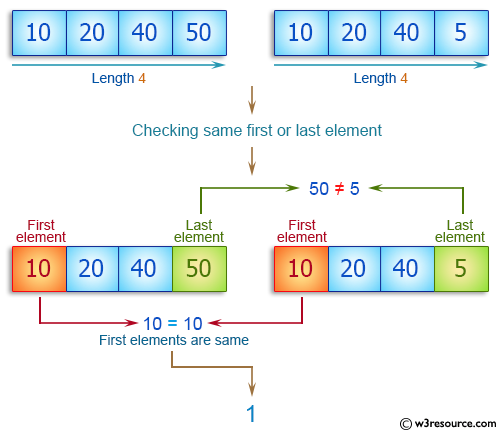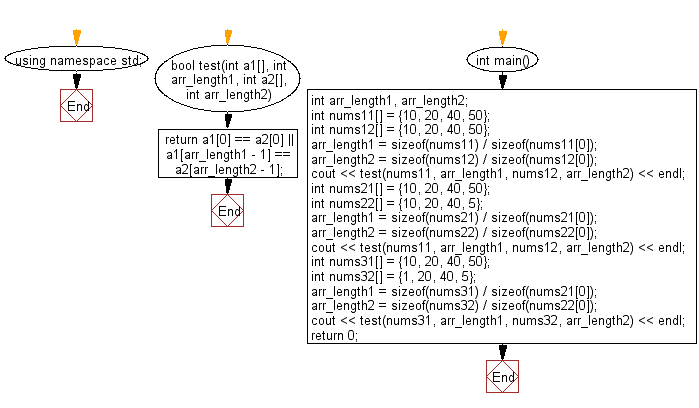﻿ C++ : Check two arrays have the same first element or last# C++ Exercises: Check two given arrays of integers of length 1 or more and return true if they have the same first element or they have the same last element

## C++ Basic Algorithm: Exercise-83 with Solution

Write a C++ program to check two given arrays of integers of length 1 or more and return true if they have the same first element or they have the same last element.

Sample Solution:

C++ Code :

``````#include <iostream>
using namespace std;

bool test(int a1[], int arr_length1, int a2[], int arr_length2)
{
return a1 == a2 || a1[arr_length1 - 1] == a2[arr_length2 - 1];
}

int main()
{
int arr_length1, arr_length2;
int nums11[] = {10, 20, 40, 50};
int nums12[] = {10, 20, 40, 50};
arr_length1 = sizeof(nums11) / sizeof(nums11);
arr_length2 = sizeof(nums12) / sizeof(nums12);
cout << test(nums11, arr_length1, nums12, arr_length2) << endl;
int nums21[] = {10, 20, 40, 50};
int nums22[] = {10, 20, 40, 5};
arr_length1 = sizeof(nums21) / sizeof(nums21);
arr_length2 = sizeof(nums22) / sizeof(nums22);
cout << test(nums11, arr_length1, nums12, arr_length2) << endl;
int nums31[] = {10, 20, 40, 50};
int nums32[] = {1, 20, 40, 5};
arr_length1 = sizeof(nums31) / sizeof(nums21);
arr_length2 = sizeof(nums32) / sizeof(nums22);
cout << test(nums31, arr_length1, nums32, arr_length2) << endl;
return 0;
}
``````

Sample Output:

```1
1
0
```

Pictorial Presentation:Flowchart:C++ Code Editor:

Contribute your code and comments through Disqus.

What is the difficulty level of this exercise?

﻿